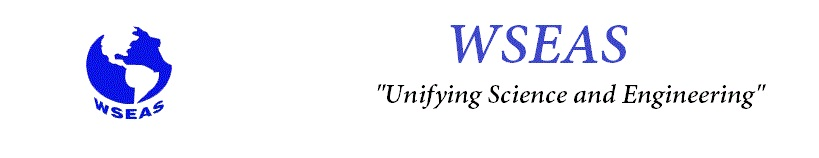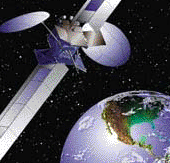Main Page of the Journal                                                          Main Page of the WSEAS October 2007, November 2007, December 2007, 2008 WSEAS TRANSACTIONS on MATHEMATICS Issue 11, Volume 6, November 2007 Print ISSN: 1109-2769 E-ISSN: 2224-2880 Title of the Paper:  A Fuzzy Statistics based Method for Mining Fuzzy Correlation Rules DOWNLOAD FULL PDF Authors: Nancy P. Lin, Hung-Jen Chen, Hao-En Chueh, Wei-Hua Hao, Chung-I Chang Abstract: Mining fuzzy association rules is the task of finding the fuzzy itemsets which frequently occur together in large fuzzy dataset, but most proposed methods may identify a fuzzy rule with two fuzzy itemsets as interesting when, in fact, the presence of one fuzzy itemsets in a record does not imply the presence of the other one in the same record. To prevent generating this kind of misleading fuzzy rule, in this paper, we construct a new method for finding relationships between fuzzy itemsets based on fuzzy statistics, and the generated rules are called fuzzy correlation rules. In our method, a fuzzy correlation analysis which can show us the strength and the type of the linear relationship between two fuzzy itemsets is used. By using thus fuzzy statistics analysis, the fuzzy correlation rules with the information about that two fuzzy not only frequently occur together in same records but also are related to each other can be generated. Keywords: Fuzzy association rules, Fuzzy itemsets, Fuzzy statistics, Fuzzy correlation analysis, Linear relationship, Fuzzy correlation rules Title of the Paper:  Convergence of the Collocation Methods for Singular Integrodifferential Equations in Lebesgue Spaces DOWNLOAD FULL PDF Authors: Iurie Caraus and Nikos E. Mastorakis Abstract: In this article, the numerical schemes of collocation methods for an approximate solution of singular integro- differential equations with kernels of Cauchy type are explained. The equations are defined on arbitrary smooth closed contours. The theoretical background of collocations methods in Lebesgue spaces is obtained. Keywords: singular integro-differential equations, collocation methods.